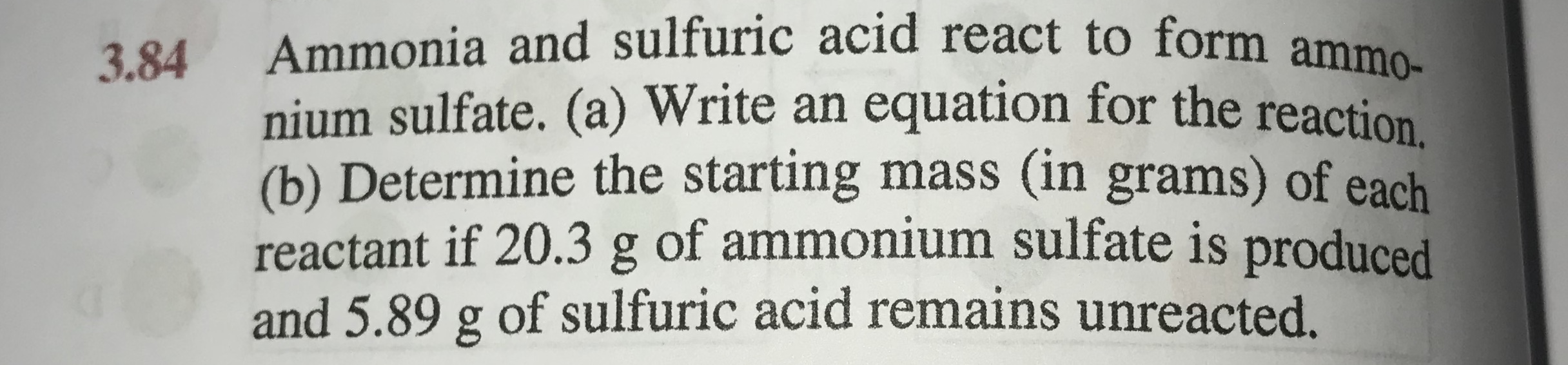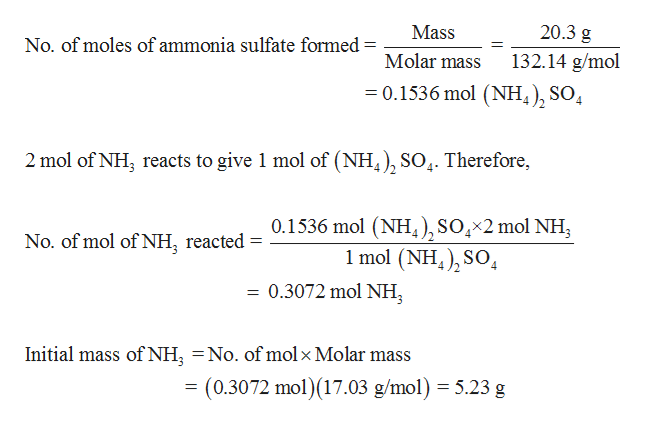Ammonia and sulfuric acid react to form ammonium sulfate. (a) Write an equation for the reaction(b) Determine the starting mass (in grams) of eachreactant if 20.3 g of ammonium sulfate is producedand 5.89 g of sulfuric acid remains unreacted.3.84

Questionhelp_outlineImage TranscriptioncloseAmmonia and sulfuric acid react to form ammo nium sulfate. (a) Write an equation for the reaction (b) Determine the starting mass (in grams) of each reactant if 20.3 g of ammonium sulfate is produced and 5.89 g of sulfuric acid remains unreacted. 3.84 fullscreen
Step 1

(a)

Balanced equation for the given reaction follows as,

Step 2

(b)

Ammonia is the limiting reagent in the given reaction. Mass ...help_outlineImage Transcriptionclose20.3 g Mass No. of moles of ammonia sulfate formed 132.14 g/mol Molar mass 0.1536 mol (NH4), SO4 2 mol of NH, reacts to give 1 mol of (NH), SO4. Therefore, 0.1536 mol (NH4), SO,*2 mol NH 1 mol (NH S04 No. of mol of NH, reacted 0.3072 mol NH Initial mass of NH No. of mol x Molar mass (0.3072 mol) (17.03 g/mol) 5.23 g fullscreen

Want to see the full answer?

See Solution

Want to see this answer and more?

Our solutions are written by experts, many with advanced degrees, and available 24/7

See Solution
Tagged in

Chemistry